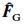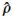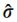# Symbols for NMR

SymbolMeaningSymbolMeaning
Roman alphabet
a or AHyperfine (electron-nucleus) coupling constantAql,mThe mth component of an irreducible tensor of order l representing the nuclear spin operator for an interaction of type q
BMagnetic Field (strictly the magnetic flux density or magnetic induction)B0Static magnetic field of an NMR spectrometer
B1,B2Radiofrequency magnetic fields associated with frequencies ν1, ν2BLLocal magnetic field (components BxL. ByL, BzL) of random field or dipolar origin
CSpin-rotation interaction tensor
CxSpin-rotation coupling constant of nuclide X
DDipolar interaction tensorDDipolar coupling constant between
two nuclei (say 1 and 2),
0/4π)γ1γ2(h/2π)r12-3in frequency units (footnote 1).
DCNuclear receptivity relative to that of the carbon-13 nucleus
DPNuclear receptivity relative to that of
the proton (hydrogen-1 nucleus)
EElectric field strength
FSpectral width
F1, F2 (or f1,f2The two frequency dimensions of a two-dimensional spectrum
(use F3 etc., for higher orders)FGMagnetic quantum number associated withgNuclear or electronic g factor (Landé splitting factor)
HijElement of matrix representation of Hamiltonian operator (in energy units) ; superscripts indicate the nature of the operator
ÎjNuclear spin operator for nucleus j (components Îjx, Îjy, Îjz)Îj+, Îj-'Raising' and 'lowering' spin operators for nucleus j
IjMagnetic quantum number associated with ÎjJIndirect coupling tensor
nJNuclear spin-spin coupling constant through n bonds (usually given in frequency units). Parentheses may be
used (for example) to indicate the species of nuclei coupled, e.g. J(13C, 1H) or, additionally, the coupling path, e.g. J(POCF). Where no ambiguity arises, the elements involved can be, alternatively, given as subscripts, e.g. JCH. The nucleus of higher mass should be given first
J(ω)Spectral density of fluctuations at angular frequency ω. Subscripts and superscripts to J may be used to indicate
the relevant quantum number change (0, 1 or 2) or the order and
component of the relevant tensor quantities.
nKReduced nuclear spin-spin coupling constant (see the notes concerning nJ), Kjk= 4π2Jjk/hγjγkLAngular momentum
mjEigenvalue of Îjz (magnetic component quantum number) (footnote 2)mtotTotal magnetic component quantum
number for a spin system (eigenvalue
of ΣjÎjz)(footnote 2)
mtot(X)Total magnetic component quantum number for X-type nuclei (footnote 2)M0Equilibrium macroscopic magnetization per volume of a spin system in the presence of B0
MX, MY, MZComponents of macroscopic magnetization per volume.Mnnth moment of spectrum (M2 = second moment, etc.)
nα, nβPopulations of the α and β spin statesNTotal number of nuclei of a given type per volume in the sample
qElectric field gradient tensor in units of the elementary charge (principal components qxx, qyy, qzz) (see also V)QeQ is the nuclear quadrupole moment,
where e is the elementary charge
RX1Spin-lattice (longitudinal) relaxation rate constant for nucleus X
RX2Spin-spin (transverse) relaxation rate constant for nucleus X
RXSpin-lattice relaxation rate constant in the rotating frame for nucleus X
SSignal intensityElectron (or, occasionally, nuclear) spin operator; cf. Ît1, t2Time dimensions for two-dimensional NMR
TCCoalescence temperature for signals in an NMR spectrum
TX1Spin-lattice (longitudinal) relaxation
time of the X nucleus (further subscripts
refer to the relaxation
mechanism)
TX2Spin-spin (transverse) relaxation time of the X nucleus (further subscripts refer to the relaxation mechanism)T*2Net dephasing time for MX or My (including contribution from magnetic field inhomogeneity)
TXSpin-lattice relaxation time of the X nucleus in the frame of reference rotating with B1
TdPulse (recycle) delay
TacAcquisition timeT(l,m)qThe mth component of an irreducible tensor of order l representing the strength of an interaction of type q
VElectric field gradient tensor. V = eq,
where e is the elementary charge
Vα,βElements of Cartesian electric field gradient tensor
W0, W1,W2Relaxation rate constants (transition
probabilities per time) between energy
levels differing by 0, 1, and 2 (respectively) in mtot especially, but not uniquely, for systems of two spin 1/2 nuclei
WrTransition probability between spin states r and s
Greek alphabet
αNuclear spin wavefunction (eigenfunction of Îjz) for the mI=+1/2 state of a spin-1/2 nucleusαEThe Ernst angle (for optimum sensitivity)
βNuclear spin wavefunction (eigenfunction of Îjz) for the mI=-1/2 state of a spin-1/2 nucleusγXMagnetogyric ratio of nucleus X
δXChemical shift (for the resonance) of nucleus of element X (positive when the sample resonates to high frequency of the reference). Usually in ppm (footnote 3). Further information regarding solvent, references or nucleus of interest may be given by superscripts or subscripts or in parentheses.ΔnPopulation difference between nuclear
states (Δn0 Boltzmann equilibrium)
ΔδChange or difference in δΔν1/2Full width in frequency units of a resonance line at half-height
ΔσAnisotropy in σ [Δσ = σzz - 1/2(σxx + σyy] (footnote 4). (see also ζΔχ(i) Susceptibility anisotropy (Δχ = χ|| - χ
(II) difference in electronegativities
ε0Permittivity of a vacuum
ζAnisotropy in shielding (footnote 4), expressed as σzz - σiso. (see also Δσ)
η(i) Nuclear Overhauser enhancement (so that the nuclear Overhauser effect is 1 + η);
(ii) tensor asymmetry factor (e.g. in σ);
(iii) viscosity
&theta:Angle, especially for that between a given vector and B0μ(i) Magnetic dipole moment (component μz along B0);
(ii) electric dipole moment
σ0Permeability of a vacuumσBBohr magneton
σNNuclear magnetonνjLarmor precession frequency of nucleus j (usually given in MHz)
ν0(i) Spectrometer operating frequency;
(ii) Larmor precession frequency (general, or of bare nucleus)
ν1Frequency of 'observing' RF magnetic Field B1 (to be distinguished from its strength, γB1, for which the symbol Ω1
is recommended)
ν2Frequency of 'irradiating' RF magnetic Field B2 (to be distinguished from its strength, γB2, for which the symbol Ω2
is recommended)
ΞxResonance frequency for the nucleus of element X in a magnetic Field such
that the protons in tetramethylsilane
(TMS) resonate at exactly 100 MHz
ρDensity matrixDensity operator
ρijElement of matrix representation ofσShielding tensor (footnotes 5 and 6)
σjIsotropic) shielding constant of nucleus j. Usually given in ppm. Subscripts may alternatively indicate contributions to σσ||, σComponents of shielding tensor σ parallel and perpendicular to the symmetry axis (axially-symmetric case)
(footnote 5)Reduced density operator
τ(i) Time between RF pulses (general symbol)
(ii) lifetime in dynamic NMR usage
τcCorrelation time for molecular-level motion, especially for isotropic molecular tumblingτdDwell time
τnullRecovery time sufficing to give zero signal after a 180° pulseτpPulse duration
τscCorrelation time for relaxation by the scalar mechanismτsrCorrelation time for spin-rotation relaxation
τ||, τperpCorrelation times for molecular tumbling parallel and perpendicular to the
symmetry axis (axially symmetric case)
Χ(i) Magnetic susceptibility (footnote 7) ;
(ii) nuclear quadrupole coupling constant (Χ = e2qzzQ/h)
ωj,
ω0,
ω1,
ω2
As for νj, ν0, ν1, ν2 but for angular frequenciesΩSpan of a Tensor
Ω1, Ω2R.f. magnetic Fields, expressed in angular frequency units for a nucleus of magnetogyric ratio γ (Ω1 = -γB1, Ω2 = -γB2
Magnetic Resonance in Chemoistry, Vol. 36, 145-149 (1998)
Parameters and Symbols for Use in Nuclear Magnetic Resonance (IUPAC Recommendations 1997)

1Note that confusion might arise when the so-called alphabet expansion is used for D, since this includes a term D which is not the dipolar coupling constant.
2M rather than m is frequently recommended, but most NMR practitioners use m so as to avoid confusion with magnetization.
3Whereas earlier IUPAC recommendations give a definition of δ which requires that the “unit" ppm is not stated when values are quoted, this is largely ignored and a change of recommendation is under consideration.
4ζ = 2Δδ/3
5The symbols σ (and related terms for components), σj, σ||, σ should refer to shielding on absolute scale (for theoretical work). For shielding relative to a reference, symbols such as σ|| - &sigmaref should be used.
6 For tensors, doubled subscript capital letters X, Y and Z should generally be used for principal components, e.g. σXX, σYY and σ ZZ for shielding. Alternatively, numerical subscripts may be used (e.g. σ11, σ22, σ33).
7 The symbol κ may also be used for magnetic susceptibility, some authors reserving χ for unrationalized units.